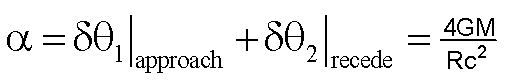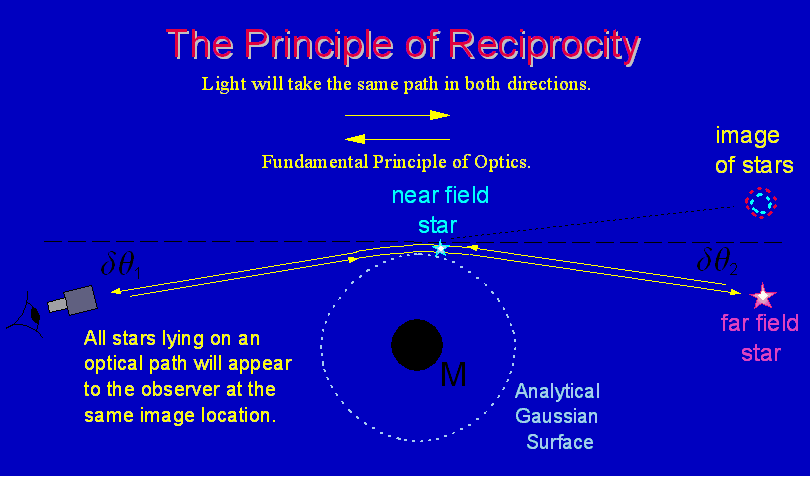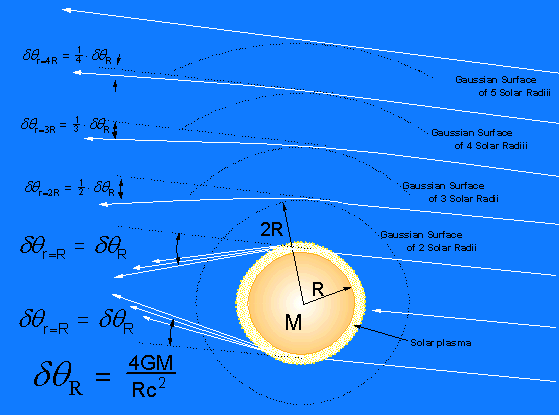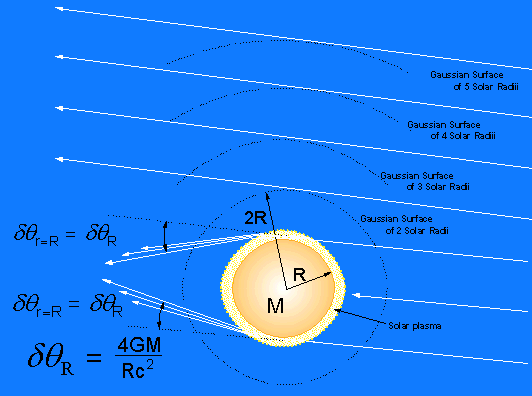Significant Findings Principle of Reciprocity and Gauss's Law of Gravity applied to Einstein Ring and Solar Light Bending   From above, the total accumulative effect is againFrom the general symmetry assumption of General Relativity and the principle of reciprocity we can see that the accumulative effect is simply the sum two equal lensing effects on approach and on receding.Fig 3 Fundamental Principle of Optical Reciprocity of the Light Ray Illustrated Regardless what one's position on gravitational lensing of light might be, the fundamental principle of optical reciprocity must hold. This is a given. The principle of optical reciprocity simply states and dictates that any light ray or a photon of light must take the very same path in either direction between the source and the observer. This is depicted in Fig 3. Historically, the effect of light bending due to gravitation has been noted only at the thin plasma rim of the sun's atmosphere.  This is a firm and well founded observational fact. Fig 4A illustrates the theoretical light bending effect of the sun, as predicted by General Relativity, which should be observed in the vacuum space far above the solar plasma rim as well as within the solar plasma rim itself. Note that the predicted light bending effect in the vacuum space far above the rim of the solar plasma; at various radii of analytical Gaussian spherical surfaces concentric to the center of the sun, is not observed at all by modern technical means.Fig 4A Gravitational light bending of the sun as function of integer solar Gaussian Surface radii in vacuum space above the rim as predicted by General Relativity (NOT OBSERVED)Fig 4B Gravitational light bending of the sun as function of integer solar Gaussian Surface radii as predicted by a Pure Classical Alternative to Relativity (OBSERVED)   Fig 4B illustrates the currently observed light bending effect of the sun at various radii of analytical Gaussian surfaces concentric to the center of the sun. A Pure Classical Alternative to General Relativity predicts the very same numerical value of light bending due to an indirect interaction between gravitation and electromagnetism in interfering media. Supporting observational evidence for this alternative is seen in the clear absence of lensing effects in the vacuum space far above the rim of the solar plasma.